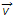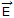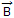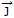# Electronics and Communication Engineering - Electromagnetic Field Theory

6.
Refraction of electromagnetic waves occurs when they
pass through a small slot in a conducting plane
pass into a medium of different dielectric constant
are polarized at right angles to the direction of propagation
encounter a perfectly conduction surface
Explanation:
No answer description is available. Let's discuss.

7.
The impedance inversion may be objected with
a short-circuited stub
an open-circuited stub
a half-wave line
a quarter-wave line
Explanation:
No answer description is available. Let's discuss.

8.
The force acting on a charge q moving with velocityin a region of electric fieldand magnetic fieldis
r+xq(+x)
q(+x)
q
Explanation:
No answer description is available. Let's discuss.

9.
An electromagnetic wave incidents on a perfect insulator at an angle. If the wave is not entirely parallel polarized, then
the reflected wave will be entirely polarized parallel to the plane of incidence
there will be no reflection
the reflected wave will be entirely polarized perpendicular to the plane of incidence
any of the above